Most Affordable JEE | NEET | 8,9,10 Preparation by Kota's Top IITian Doctor Faculties

# NCERT Solutions for Class 11 Physics chapter 6 - Work, Energy and Power - Free PDF Download`
Hey, are you a class 11 student and looking for ways to download NCERT Solutions for Class 11 Physics chapter 6 "Work, Energy and Power"? If yes. Then read this post till the end.

In this article, we have listed NCERT solution for class 11 Physics Chapter 6 in PDF that are prepared by Kota’s top IITian’s Faculties by keeping Simplicity in mind.

If you want to learn and understand class 11 Physics chapter 6 "Work, Energy and Power" in an easy way then you can use these solutions PDF.

NCERT Solutions helps students to Practice important concepts of subjects easily. Class 11 Physics solutions provide detailed explanations of all the NCERT questions that students can use to clear their doubts instantly.

If you want to score high in your class 11 Physics Exam then it is very important for you to have a good knowledge of all the important topics, so to learn and practice those topics you can use eSaral NCERT Solutions.

So, without wasting more time Let’s start.

### Download The PDF of NCERT Solutions for Class 11 Physics Chapter 6 "Work, Energy and Power"

Question 1: The sign of work done by a force on a body is important to understand. State carefully if the following quantities are positive or negative:
(a) work done by a man in lifting a bucket out of a well by means of a rope tied to the bucket.
(b) work done by gravitational force in the above case,
(c) work done by friction on a body sliding down an inclined plane,
(d) work done by an applied force on a body moving on a rough horizontal plane with uniform velocity,
(e) work done by the resistive force of air on a vibrating pendulum in bringing it to rest.

Solution.
(a) Positive
In the given case, force and displacement are in the same direction. Hence, the sign of work done is positive. In this case, the work is done on the bucket.
(b) Negative
In the given case, the direction of force (vertically downward) and displacement (vertically upward) are opposite to each other. Hence, the sign of work done is negative.
(c) Negative
Since the direction of frictional force is opposite to the direction of motion, the work done by frictional force is negative in this case.
(d) Positive
Here the body is moving on a rough horizontal plane. Frictional force opposes the motion of the body. Therefore, in order to maintain a uniform velocity, a uniform force must be applied to the body. Since the applied force acts in the direction of motion of the body, the work done is positive.
(e) Negative
The resistive force of air acts in the direction opposite to the direction of motion of the pendulum. Hence, the work done is negative in this case.

Question 2:
A body of mass 2 kg initially at rest moves under the action of an applied horizontal force of 7 N on a table with coefficient of kinetic friction = 0.1.
Compute the
(a) work done by the applied force in 10 s,
(b) work done by friction in 10 s,
(c) work done by the net force on the body in 10 s,
(d) change in kinetic energy of the body in 10 s,

Solution.
Mass of the body, $m=2 \mathrm{~kg}$
Applied force, $F=7 \mathrm{~N}$
Coefficient of kinetic friction, $\mu=0.1$
Initial velocity, $u=0$
Time, $t=10 \mathrm{~s}$
The acceleration produced in the body by the applied force is given by Newton's second law of motion as:
$a^{\prime}=\frac{F}{m}=\frac{7}{2}=3.5 \mathrm{~m} / \mathrm{s}^{2}$
Frictional force is given as:
$f=\mu m g$
$=0.1 \times 2 \times 9.8=-1.96 \mathrm{~N}$ The acceleration produced by the frictional force:
$a^{\prime \prime}=-\frac{1.96}{2}=-0.98 \mathrm{~m} / \mathrm{s}^{2}$
Total acceleration of the body:
$a=a^{\prime}+a^{\prime \prime}$
$=3.5+(-0.98)=2.52 \mathrm{~m} / \mathrm{s}^{2}$
The distance travelled by the body is given by the equation of motion:
$s=u t+\frac{1}{2} a t^{2}$
$=0+\frac{1}{2} \times 2.52 \times(10)^{2}=126 \mathrm{~m}$
(a) Work done by the applied force, $W_{\mathrm{a}}=\mathrm{F} \times \mathrm{s}=7 \times 126=882 \mathrm{~J}$
(b) Work done by the frictional force, $W_{\mathrm{f}}=F \times s=-1.96 \times 126=-247 \mathrm{~J}$
(c) Net force $=7+(-1.96)=5.04 \mathrm{~N}$
Work done by the net force, $W_{\text {net }}=5.04 \times 126=635 \mathrm{~J}$
(d) From the first equation of motion, final velocity can be calculated as:
$v=u+a t$
$=0+2.52 \times 10=25.2 \mathrm{~m} / \mathrm{s}$
Change in kinetic energy $=\frac{1}{2} m v^{2}-\frac{1}{2} m u^{2}$
$=\frac{1}{2} \times 2\left(v^{2}-u^{2}\right)=(25.2)^{2}-0^{2}=635 \mathrm{~J}$

Question 3:
Given in Fig. $6.11$ are examples of some potential energy functions in one dimension. The total energy of the particle is indicated by a cross on the ordinate axis. In each case, specify the regions, if any, in which the particle cannot be found for the given energy. Also, indicate the minimum total energy the particle must have in each case. Think of simple physical contexts for which these potential energy shapes are relevant.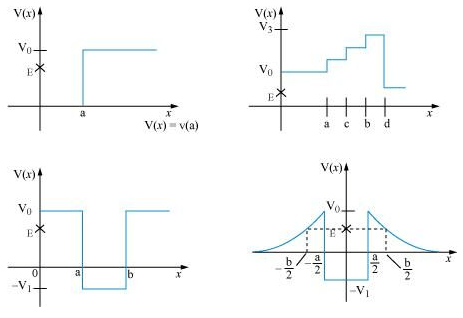Solution.
(a) $x>a ; 0$
Total energy of a system is given by the relation:
$E=$ P.E. $+K$. E.
$\therefore K \cdot E .=E-P . E$
Kinetic energy of a body is a positive quantity. It cannot be negative. Therefore, the particle will not exist in a region where K.E. becomes negative.
In the given case, the potential energy $\left(V_{0}\right)$ of the particle becomes greater than total energy $(E)$ for $x>a$. Hence, kinetic energy becomes negative in this region. Therefore, the particle will not exist is this region. The minimum total energy of the particle is zero.
(b) All regions
In the given case, the potential energy $\left(V_{0}\right)$ is greater than total energy (E) in all regions. Hence, the particle will not exist in this region.
(c) $x>a$ and $x In the given case, the condition regarding the positivity of$\mathrm{K.E}$. is satisfied only in the region between$x>a$and$x The minimum potential energy in this case is $-V_{1}$. Therfore, K.E. $=E-\left(-V_{1}\right)=E+V_{1}$. Therefore, for the positivity of the kinetic energy, the totaol energy of the particle must be greater than $-V_{1}$. So, the minimum total energy the particle must have is $-V_{1}$.
(d) $-\frac{b}{2} In the given case, the potential energy$\left(V_{0}\right)$of the particle becomes greater than the total energy$(E)$for$-\frac{b}{2} The minimum potential energy in this case is $-V_{1}$. Therfore, K.E. $=E-\left(-V_{1}\right)=E+V_{1}$. Therefore, for the positivity of the kinetic energy, the totaol energy of the particle must be greater than $-V_{1}$. So, the minimum total energy the particle must have is $-V_{1}$.

Question 4 :
The potential energy function for a particle executing linear simple harmonic motion is given by $V(x)=k x^{2} / 2$, where $k$ is the force constant of the oscillator. For $k=0.5 \mathrm{~N} \mathrm{~m}^{-1}$, the graph of $V(x)$ versus $x$ is shown in Fig. $6.12$. Show that a particle of total energy $1 \mathrm{~J}$ moving under this potential must 'turn back' when it reaches $x=\pm 2 \mathrm{~m}$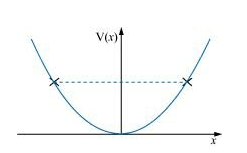Solution.
Total energy of the particle, $E=1 \mathrm{~J}$
Force constant, $k=0.5 \mathrm{~N} \mathrm{~m}^{-1}$
Kinetic energy of the particle, $\mathrm{K}=\frac{1}{2} m v^{2}$
According to the conservation law:
$E=V+K$
$1=\frac{1}{2} k x^{2}+\frac{1}{2} m v^{2}$
At the moment of 'turn back', velocity (and hence $K$ ) becomes zero.
$\therefore 1=\frac{1}{2} k x^{2}$
$\frac{1}{2} \times 0.5 x^{2}=1$
$x^{2}=4$
$x=\pm 2$
Hence, the particle turns back when it reaches $x=\pm 2 \mathrm{~m}$.

Question 5:
(a) The casing of a rocket in flight burns up due to friction. At whose expense is the heat energy required for burning obtained? The rocket or the atmosphere?
(b) Comets move around the sun in highly elliptical orbits. The gravitational force on the comet due to the sun is not normal to the comet’s velocity in general. Yet the work done by the gravitational force over every complete orbit of the comet is zero. Why?
(c) An artificial satellite orbiting the earth in very thin atmosphere loses its energy gradually due to dissipation against atmospheric resistance, however small. Why then does its speed increase progressively as it comes closer and closer to the earth?
(d) In Fig. the man walks 2 m carrying a mass of 15 kg on his hands. In Fig. 6.13(ii), he walks the same distance pulling the rope behind him. The rope goes over a pulley, and a mass of 15 kg hangs at its other end. In which case is the work done greater?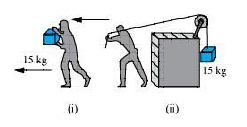Fig.

Solution.
(a) Rocket
The burning of the casing of a rocket in flight (due to friction) results in the reduction of the mass of the rocket.
According to the conservation of energy:
Total Energy (T.E.) = Potential energy (P.E.) + Kinetic energy (K.E.)
$=m \mathrm{~g} h+\frac{1}{2} m v^{2}$\ v The reduction in the rocket’s mass causes a drop in the total energy. Therefore, the heat energy required for the burning is obtained from the rocket. \
(b) Gravitational force is a conservative force. Since the work done by a conservative force over a closed path is zero, the work done by the gravitational force over every complete orbit of a comet is zero.
(c) When an artificial satellite, orbiting around earth, moves closer to earth, its potential energy decreases because of the reduction in the height. Since the total energy of the system remains constant, the reduction in P.E. results in an increase in K.E. Hence, the velocity of the satellite increases. However, due to atmospheric friction, the total energy of the satellite decreases by a small amount.
(d) In the second case
Case (i)
Mass, m = 15 kg
Displacement, s = 2 m
Work done, $W=F s \cos \theta$
Where, $\theta=$ Angle between force and displacement]
$=m g s \cos \theta=15 \times 2 \times 9.8 \cos 90^{\circ}$
$=m g s \cos \theta=15 \times 2 \times 9.8 \cos 90^{\circ}$
$=0 \quad\left(\because \cos 90^{\circ}=0\right)$
Case (ii)
Mass, m = 15 kg \
Displacement, s = 2 m
Here, the direction of the force applied on the rope and the direction of the displacement of the rope are same.
Therefore, the angle between them, θ = 0°
Since cos 0° = 1
Work done, W = Fs cosθ = mgs
= 15 × 9.8 × 2 = 294 J
Hence, more work is done in the second case.

Question 6:
Underline the correct alternative:
(a) When a conservative force does positive work on a body, the potential energy of the body increases/decreases/remains unaltered.\
(b) Work done by a body against friction always results in a loss of its kinetic/potential energy.
(c) The rate of change of total momentum of a many-particle system is proportional to the external force/sum of the internal forces on the system.
(d) In an inelastic collision of two bodies, the quantities which do not change after the collision are the total kinetic energy/total linear momentum/total energy of the system of two bodies.

Solution.
(a) Decreases
(b) Kinetic energy
(c) External force
(d) Total linear momentum
Explanation:
(a) A conservative force does a positive work on a body when it displaces the body in the direction of force. As a result, the body advances toward the centre of force. It decreases the separation between the two, thereby decreasing the potential energy of the body.
(b) The work done against the direction of friction reduces the velocity of a body. Hence, there is a loss of kinetic energy of the body.
(c) Internal forces, irrespective of their direction, cannot produce any change in the total momentum of a body. Hence, the total momentum of a many- particle system is proportional to the external forces acting on the system.
(d) The total linear momentum always remains conserved whether it is an elastic collision or an inelastic collision.

Question 7:
State if each of the following statements is true or false. Give reasons for your answer.
(a) In an elastic collision of two bodies, the momentum and energy of each body is conserved.
(b) Total energy of a system is always conserved, no matter what internal and external forces on the body are present.
(c) Work done in the motion of a body over a closed loop is zero for every force in nature.
(d) In an inelastic collision, the final kinetic energy is always less than the initial kinetic energy of the system.

Solution.
(a) False
(b) False
(c) False
(d) True
Explanation:
(a) In an elastic collision, the total energy and momentum of both the bodies, and not of each individual body, is conserved.
(b) Although internal forces are balanced, they cause no work to be done on a body. It is the external forces that have the ability to do work. Hence, external forces are able to change the energy of a system.
(c) The work done in the motion of a body over a closed loop is zero for a conservation force only.
(d) In an inelastic collision, the final kinetic energy is always less than the initial kinetic energy of the system. This is because in such collisions, there is always a loss of energy in the form of heat, sound, etc.

Question 8:
(a) In an elastic collision of two billiard balls, is the total kinetic energy conserved during the short time of collision of the balls (i.e. when they are in contact)?
(b) Is the total linear momentum conserved during the short time of an elastic collision of two balls?
(c) What are the answers to (a) and (b) for an inelastic collision?
(d) If the potential energy of two billiard balls depends only on the separation distance between their centres, is the collision elastic or inelastic? (Note, we are talking here of potential energy corresponding to the force during collision, not gravitational potential energy).

Solution.
(a) No
In an elastic collision, the total initial kinetic energy of the balls will be equal to the total final kinetic energy of the balls. This kinetic energy is not conserved at the instant the two balls are in contact with each other. In fact, at the time of collision, the kinetic energy of the balls will get converted into potential energy.
(b) Yes
In an elastic collision, the total linear momentum of the system always remains conserved.
(c) No; Yes
In an inelastic collision, there is always a loss of kinetic energy, i.e., the total kinetic energy of the billiard balls before collision will always be greater than that after collision.
The total linear momentum of the system of billiards balls will remain conserved even in the case of an inelastic collision.
(d) Elastic
In the given case, the forces involved are conservation. This is because they depend on the separation between the centres of the billiard balls. Hence, the collision is elastic.

Question 9:
A body is initially at rest. It undergoes one-dimensional motion with constant acceleration. The power delivered to it at time $t$ is proportional to
(i) $t^{\frac{1}{2}}$
(ii) $t$ (iii) $t^{\frac{3}{2}}$
(iv) $t^{2}$
Answer: (ii) $t$
Mass of the body $=m$
Acceleration of the body $=a$
Using Newton's second law of motion, the force experienced by the body is given by the equation:
$F=m a$
Both $m$ and $a$ are constants. Hence, force $F$ will also be a constant.
$F=m a=$ Constant $\ldots$ (i)
For velocity $v$, acceleration is given as,
$a=\frac{d v}{d t}=$ Constant
$d v=$ Constant $\times d t$
$v=\alpha t \quad \ldots$
Where, $\alpha$ is another constant
$v \propto t \quad \ldots($ iii $)$
Power is given by the relation:
$P=F \cdot V$
Using equations (i) and (iii), we have:
$P \propto t$
Hence, power is directly proportional to time.

Question 10:
A body is moving unidirectionally under the influence of a source of constant power. Its displacement in time $t$ is proportional to
(i) $t^{\frac{1}{2}}$
(ii) $t$ (iii) $t^{\frac{3}{2}}$
(iv) $t^{2}$

Solution.
(iii) $t^{\frac{3}{2}}$
Power is given by the relation:
$P=F v$
$=m a v=m v \frac{d v}{d t}=$ Constant (say, $\left.k\right)$
$\therefore v d v=\frac{k}{m} d t$
Integrating both sides:
$\frac{v^{2}}{2}=\frac{k}{m} t$
$v=\sqrt{\frac{2 k t}{m}}$
For displacement $x$ of the body, we have:
$v=\frac{d x}{d t}=\sqrt{\frac{2 k}{m}} t^{\frac{1}{2}}$\
$d x=k^{\prime} t^{\frac{1}{2}} d t$
Where $k^{\prime}=\sqrt{\frac{2 k}{3}}=$ New constant
On integrating both sides, we get:
$x=\frac{2}{3} k^{\prime} t^{\frac{3}{2}}$ ]
$\therefore x \propto t^{\frac{3}{2}}$

Question 6.11:
A body constrained to move along the z-axis of a coordinate system is subject to a constant force $\mathbf{F}$ given by $\mathbf{F}=-\hat{\mathbf{i}}+2 \hat{\mathbf{j}}+3 \hat{\mathbf{k}} \mathrm{N}$
Where $\hat{\mathbf{i}}, \hat{\mathbf{j}}, \hat{\mathbf{k}}$ are unit vectors along the $x-, y$ - and $z$ -axis of the system respectively. What is the work done by this force in moving the body a distance of $4 \mathrm{~m}$ along the $z$ -axis?

Solution.
Force exerted on the body, $\mathbf{F}=-\hat{\mathbf{i}}+2 \hat{\mathbf{j}}+3 \hat{\mathbf{k}} \mathrm{N}$
Displacement, $s=4 \hat{\mathbf{k}} \mathrm{m}$
Work done, $W=$ F.s
$=(-\hat{\mathbf{i}}+2 \hat{\mathbf{j}}+3 \hat{\mathbf{k}}) \cdot(4 \hat{\mathbf{k}})$
$=0+0-3 \times 4$
$=12 \mathrm{~J}$
Hence, $12 \mathrm{~J}$ of work is done by the force on the body.

Question 12.
An electron and a proton are detected in a cosmic ray experiment, the first with kinetic energy $10 \mathrm{keV}$, and the second with $100 \mathrm{keV}$. Which is faster, the electron or the proton? Obtain the ratio of their speeds. (electron mass $=9.11 \times 10^{-31} \mathrm{~kg}$, proton mass $=1.67 \times 10^{-27} \mathrm{~kg}, 1 \mathrm{eV}=1.60 \times 10^{-19} \mathrm{~J}$ ).

Solution.
Electron is faster; Ratio of speeds is $13.54: 1$
Mass of the electron, $m_{\mathrm{e}}=9.11 \times 10^{-31} \mathrm{~kg}$
Mass of the proton, $m_{\mathrm{p}}=1.67 \times 10^{-27} \mathrm{~kg}$
Kinetic energy of the electron, $E_{\mathrm{Ke}}=10 \mathrm{keV}=10^{4} \mathrm{eV}$
$=10^{4} \times 1.60 \times 10^{-19}$
$=1.60 \times 10^{-15} \mathrm{~J}$
Kinetic energy of the proton, $E_{K p}=100 \mathrm{keV}=10^{5} \mathrm{eV}=1.60 \times 10^{-14} \mathrm{~J}$
For the velocity of an electron $v_{e}$, its kinetic energy is given by the relation:
$E_{\mathrm{Kc}}=\frac{1}{2} m v_{\mathrm{c}}^{2}$
$\therefore v_{\mathrm{e}}=\sqrt{\frac{2 \times E_{\mathrm{Ke}}}{m}}$
$=\sqrt{\frac{2 \times 1.60 \times 10^{-15}}{9.11 \times 10^{-31}}}=5.93 \times 10^{7} \mathrm{~m} / \mathrm{s}$
For the velocity of a proton $v_{\mathrm{p}}$, its kinetic energy is given by the relation:
$E_{\mathrm{Kp}}=\frac{1}{2} m v_{\mathrm{p}}^{2}$
$v_{\mathrm{p}}=\sqrt{\frac{2 \times E_{\mathrm{Kp}}}{m}}$
$\therefore v_{\mathrm{p}}=\sqrt{\frac{2 \times 1.6 \times 10^{-14}}{1.67 \times 10^{-27}}}=4.38 \times 10^{6} \mathrm{~m} / \mathrm{s}$
Hence, the electron is moving faster than the proton.
The ratio of their speeds:
$\frac{v_{\mathrm{c}}}{v_{\mathrm{p}}}=\frac{5.93 \times 10^{7}}{4.38 \times 10^{6}}=13.54: 1$

Question 13:
A rain drop of radius 2 mm falls from a height of 500 m above the ground. It falls with decreasing acceleration (due to viscous resistance of the air) until at half its original height, it attains its maximum (terminal) speed, and moves with uniform speed thereafter. What is the work done by the gravitational force on the drop in the first and second half of its journey? What is the work done by the resistive force in the entire journey if its speed on reaching the ground is 10 m s–1?

Solution.
Radius of the rain drop, $r=2 \mathrm{~mm}=2 \times 10^{-3} \mathrm{~m}$
Volume of the rain drop, $V=\frac{4}{3} \pi r^{3}$
$=\frac{4}{3} \times 3.14 \times\left(2 \times 10^{-3}\right)^{3} \mathrm{~m}^{-3}$
Density of water, $\rho=10^{3} \mathrm{~kg} \mathrm{~m}^{-3}$
Mass of the rain drop, $m=\rho V$
$=\frac{4}{3} \times 3.14 \times\left(2 \times 10^{-3}\right)^{3} \times 10^{3} \mathrm{~kg}$
Gravitational force, $F=m \mathrm{~g}$
$=\frac{4}{3} \times 3.14 \times\left(2 \times 10^{-3}\right)^{3} \times 10^{3} \times 9.8 \mathrm{~N}$
The work done by the gravitational force on the drop in the first half of its journey:
$W_{1}=F_{S}$
$=\frac{4}{3} \times 3.14 \times\left(2 \times 10^{-3}\right)^{3} \times 10^{3} \times 9.8 \times 250$
$=0.082 \mathrm{~J}$
This amount of work is equal to the work done by the gravitational force on the drop in the second half of its journey, i.e., $W_{\|,}=0.082 \mathrm{~J}$
As per the law of conservation of energy, if no resistive force is present, then the total energy of the rain drop will remain the same.
$\therefore$ Total energy at the top
$E_{\mathrm{T}}=m g h+0$
$=\frac{4}{3} \times 3.14 \times\left(2 \times 10^{-3}\right)^{3} \times 10^{3} \times 9.8 \times 500 \times 10^{-5}$
$=0.164 \mathrm{~J}$
Due to the presence of a resistive force, the drop hits the ground with a velocity of $10 \mathrm{~m} / \mathrm{s}$.
$\therefore$ Total energy at the ground:
$E_{\mathrm{G}}=\frac{1}{2} m v^{2}+0$
$=\frac{1}{2} \times \frac{4}{3} \times 3.14 \times\left(2 \times 10^{-3}\right)^{3} \times 10^{3} \times 9.8 \times(10)^{2}$
$=1.675 \times 10^{-3} \mathrm{~J}$
$\therefore$ Resistive force $=E_{G}-E_{T}=-0.162 \mathrm{~J}$

Question 14.
A molecule in a gas container hits a horizontal wall with speed $200 \mathrm{~m} \mathrm{~s}^{-1}$ and angle $30^{\circ}$ with the normal, and rebounds with the same speed. Is momentum conserved in the collision? Is the collision elastic or inelastic?

Solution.
Yes; Collision is elastic
The momentum of the gas molecule remains conserved whether the collision is elastic or inelastic.
The gas molecule moves with a velocity of 200 m/s and strikes the stationary wall of the container, rebounding with the same speed.
It shows that the rebound velocity of the wall remains zero. Hence, the total kinetic energy of the molecule remains conserved during the collision. The given collision is an example of an elastic collision.

Question 15 :
A pump on the ground floor of a building can pump up water to fill a tank of volume $30 \mathrm{~m}^{3}$ in $15 \mathrm{~min}$. If the tank is $40 \mathrm{~m}$ above the ground, and the efficiency of the pump is $30 \%$, how much electric power is consumed by the pump?

Solution.
Volume of the tank, $V=30 \mathrm{~m}^{3}$
Time of operation, $t=15 \min =15 \times 60=900 \mathrm{~s}$
Height of the tank, $h=40 \mathrm{~m}$
Efficiency of the pump, $\eta=30 \%$
Density of water, $\rho=10^{3} \mathrm{~kg} / \mathrm{m}^{3}$
Mass of water, $m=\rho V=30 \times 10^{3} \mathrm{~kg}$
Output power can be obtained as:
$P_{0}=\frac{\text { Work done }}{\text { Time }}=\frac{m g h}{t}$
$=\frac{30 \times 10^{3} \times 9.8 \times 40}{900}=13.067 \times 10^{3} \mathrm{~W}$
For input power $P_{b}$, efficiency $\eta$, is given by the relation:
\begin{aligned} \eta &=\frac{P_{0}}{P_{i}}=30 \% \\ P_{i} &=\frac{13.067}{30} \times 100 \times 10^{3} \\ &=0.436 \times 10^{5} \mathrm{~W} \\ &=43.6 \mathrm{~kW} \end{aligned}

Question 16:
Two identical ball bearings in contact with each other and resting on a frictionless table are hit head-on by another ball bearing of the same mass moving initially with a speed $V$. If the collision is elastic, which of the following figure is a possible result after collision?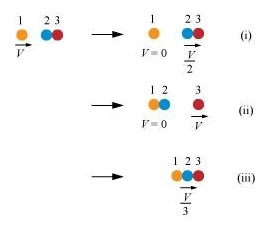Solution.
Case (ii)
It can be observed that the total momentum before and after collision in each case is constant.
For an elastic collision, the total kinetic energy of a system remains conserved before and after collision.
For mass of each ball bearing m, we can write:
Total kinetic energy of the system before collision:
$=\frac{1}{2} m V^{2}+\frac{1}{2}(2 m) 0$
$=\frac{1}{2} m V^{2}$
Hence, the kinetic energy of the system is not conserved in case (i).
Case (ii)
Total kinetic energy of the system after collision:
$=\frac{1}{2}(2 m) \times 0+\frac{1}{2} m V^{2}$
$=\frac{1}{2} m V^{2}$
Hence, the kinetic energy of the system is not conserved in case (iii).

Question 17:
The bob A of a pendulum released from 30° to the vertical hits another bob B of the same mass at rest on a table as shown in Fig. 6.15. How high does the bob A rise after the collision? Neglect the size of the bobs and assume the collision to be elastic.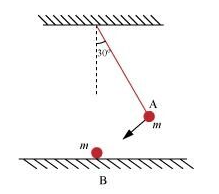Solution.
Bob A will not rise at all
In an elastic collision between two equal masses in which one is stationary, while the other is moving with some velocity, the stationary mass acquires the same velocity, while the moving mass immediately comes to rest after collision. In this case, a complete transfer of momentum takes place from the moving mass to the stationary mass.
Hence, bob A of mass m, after colliding with bob B of equal mass, will come to rest, while bob B will move with the velocity of bob A at the instant of collision.

Question 18:
The bob of a pendulum is released from a horizontal position. If the length of the pendulum is 1.5 m, what is the speed with which the bob arrives at the lowermost point, given that it dissipated 5% of its initial energy against air resistance?

Solution.
Length of the pendulum, $I=1.5 \mathrm{~m}$
Mass of the bob $=m$
Energy dissipated $=5 \%$
According to the law of conservation of energy, the total energy of the system remains constant.
At the horizontal position:
Potential energy of the bob, $E_{\mathrm{P}}=\mathrm{mg} /$
Kinetic energy of the bob, $E_{K}=0$
Total energy $=m g l \ldots$ (i)
At the lowermost point (mean position):
Potential energy of the bob, $E_{p}=0$
Kinetic energy of the bob, $E_{\mathrm{K}}=\frac{1}{2} m v^{2}$
Total energy $E_{x}=\frac{1}{2} m v^{2} \ldots$ (ii)
As the bob moves from the horizontal position to the lowermost point, $5 \%$ of its energy gets dissipated.
The total energy at the lowermost point is equal to $95 \%$ of the total energy at the horizontal point, i.e.,
$\frac{1}{2} m v^{2}=\frac{95}{100} \times m g l$
$v=\sqrt{\frac{2 \times 95 \times 1.5 \times 9.8}{100}}$
$=5.28 \mathrm{~m} / \mathrm{s}$

Question 6.19:
A trolley of mass 300 kg carrying a sandbag of 25 kg is moving uniformly with a speed of 27 km/h on a frictionless track. After a while, sand starts leaking out of a hole on the floor of the trolley at the rate of 0.05 kg s–1. What is the speed of the trolley after the entire sand bag is empty?

Solution.
The sand bag is placed on a trolley that is moving with a uniform speed of 27 km/h. The external forces acting on the system of the sandbag and the trolley is zero. When the sand starts leaking from the bag, there will be no change in the velocity of the trolley. This is because the leaking action does not produce any external force on the system. This is in accordance with Newton’s first law of motion. Hence, the speed of the trolley will remain 27 km/h.

Question 20:
A body of mass $0.5 \mathrm{~kg}$ travels in a straight line with velocity $v=a x^{\frac{3}{2}}$ where $a=5 \mathrm{~m}^{-\frac{1}{2}} \mathrm{~s}^{-1}$. What is the work done by the net force during its displacement from $x=0$ to $x=2 \mathrm{~m} ?$

Solution.
Mass of the body, $m=0.5 \mathrm{~kg}$
Velocity of the body is governed by the equation, $v=a x^{\frac{3}{2}}$ with $a=5 \mathrm{~m}^{\frac{-1}{2}} \mathrm{~s}^{-1}$
Initial velocity, $u($ at $x=0)=0$
Final velocity $v($ at $x=2 \mathrm{~m})=10 \sqrt{2} \mathrm{~m} / \mathrm{s}$
Work done, $W=$ Change in kinetic energy
$=\frac{1}{2} m\left(v^{2}-u^{2}\right)$
$=\frac{1}{2} \times 0.5\left[(10 \sqrt{2})^{2}-(0)^{2}\right]$
$=\frac{1}{2} \times 0.5 \times 10 \times 10 \times 2$
$=50 \mathrm{~J}$\

Question 21:
The blades of a windmill sweep out a circle of area $A$. (a) If the wind flows at a velocity $v$ perpendicular to the circle, what is the mass of the air passing through it in time $t ?(\mathrm{~b})$ What is the kinetic energy of the air? (c) Assume that the windmill converts $25 \%$ of the wind's energy into electrical energy, and that $A=30 \mathrm{~m}^{2}, v=36 \mathrm{~km} / \mathrm{h}$ and the density of air is $1.2$ $\mathrm{kg} \mathrm{m}^{-3}$. What is the electrical power produced?

Solution.
Area of the circle swept by the windmill $=A$
Velocity of the wind $=v$
Density of air $=\rho$
(a) Volume of the wind flowing through the windmill per $\mathrm{sec}=\mathrm{Av}$
Mass of the wind flowing through the windmill per $\mathrm{sec}=\rho A v$
Mass $m$, of the wind flowing through the windmill in time $t=\rho$ Avt
(b) Kinetic energy of air $=\frac{1}{2} m v^{2}$
$=\frac{1}{2}(\rho A v t) v^{2}=\frac{1}{2} \rho A v^{3} t$
(c) Area of the circle swept by the windmill $=A=30 \mathrm{~m}^{2}$
Velocity of the wind $=v=36 \mathrm{~km} / \mathrm{h}$
Density of air, $\rho=1.2 \mathrm{~kg} \mathrm{~m}^{-3}$\
Electric energy produced $=25 \%$ of the wind energy
$=\frac{25}{100} \times$ Kinetic energy of air
$=\frac{1}{8} \rho A v^{3} t$
$=\frac{1}{8} \frac{\rho A v^{3} t}{t}=\frac{1}{8} \rho A v^{3}$
$=\frac{1}{8} \times 1.2 \times 30 \times(10)^{3}$
$=4.5 \times 10^{3} \mathrm{~W}=4.5 \mathrm{~kW}$

Question 22:
A person trying to lose weight (dieter) lifts a $10 \mathrm{~kg}$ mass, one thousand times, to a height of $0.5 \mathrm{~m}$ each time. Assume that the potential energy lost each time she lowers the mass is dissipated. (a) How much work does she do against the gravitational force? (b) Fat supplies $3.8 \times 10^{7} \mathrm{~J}$ of energy per kilogram which is converted to mechanical energy with a $20 \%$ efficiency rate. How much fat will the dieter use up?

Solution.
(a) Mass of the weight, $m=10 \mathrm{~kg}$
Height to which the person lifts the weight, $h=0.5 \mathrm{~m}$
Number of times the weight is lifted, $n=1000$
$\therefore$ Work done against gravitational force:
$=n(m \mathrm{~g} h)$
$=1000 \times 10 \times 9.8 \times 0.5$
$=49 \times 10^{3} \mathrm{~J}=49 \mathrm{~kJ}$
(b) Energy equivalent of $1 \mathrm{~kg}$ of fat $=3.8 \times 10^{7} \mathrm{~J}$ Efficiency rate $=20 \%$
Mechanical energy supplied by the person's body:
$=\frac{20}{100} \times 3.8 \times 10^{7} \mathrm{~J}$
$=\frac{1}{5} \times 3.8 \times 10^{7} \mathrm{~J}$
Equivalent mass of fat lost by the dieter:
$=\frac{1}{\frac{1}{5} \times 3.8 \times 10^{7}} \times 49 \times 10^{3}$
$=\frac{245}{3.8} \times 10^{-4}$
$=6.45 \times 10^{-3} \mathrm{~kg}$

Question 23:
A family uses 8 kW of power. (a) Direct solar energy is incident on the horizontal surface at an average rate of 200 W per square meter. If 20% of this energy can be converted to useful electrical energy, how large an area is needed to supply 8 kW? (b) Compare this area to that of the roof of a typical house.

Solution.
(a) $200 \mathrm{~m}^{2}$
(a) Power used by the family, $P=8 \mathrm{~kW}=8 \times 10^{3} \mathrm{~W}$
Solar energy received per square metre $=200 \mathrm{~W}$
Efficiency of conversion from solar to electricity energy $=20 \%$
Area required to generate the desired electricity $=A$
As per the information given in the question, we have:
$8 \times 10^{3}=20 \% \times(A \times 200)$
$=\frac{20}{100} \times A \times 200$
$\therefore A=\frac{8 \times 10^{3}}{40}=200 \mathrm{~m}^{2}$
(b) The area of a solar plate required to generate 8 kW of electricity is almost equivalent to the area of the roof of a building having dimensions 14 m × 14 m.

Question 24:
A bullet of mass $0.012 \mathrm{~kg}$ and horizontal speed $70 \mathrm{~m} \mathrm{~s}^{-1}$ strikes a block of wood of mass $0.4 \mathrm{~kg}$ and instantly comes to rest with respect to the block. The block is suspended from the ceiling by means of thin wires. Calculate the height to which the block rises. Also, estimate the amount of heat produced in the block.

Solution.
Mass of the bullet, $m=0.012 \mathrm{~kg}$
Initial speed of the bullet, $u_{\mathrm{b}}=70 \mathrm{~m} / \mathrm{s}$
Mass of the wooden block, $M=0.4 \mathrm{~kg}$
Initial speed of the wooden block, $u_{\mathrm{B}}=0$
Final speed of the system of the bullet and the block $=v$
Applying the law of conservation of momentum:
$m u_{\mathrm{b}}+M u_{\mathrm{B}}=(m+M) v$
$0.012 \times 70+0.4 \times 0=(0.012+0.4) v$
$\therefore v=\frac{0.84}{0.412}=2.04 \mathrm{~m} / \mathrm{s}$
For the system of the bullet and the wooden block:
Mass of the system, $m^{\prime}=0.412 \mathrm{~kg}$
Velocity of the system $=2.04 \mathrm{~m} / \mathrm{s}$
Height up to which the system rises $=h$
Applying the law of conservation of energy to this system:
Potential energy at the highest point = Kinetic energy at the lowest point
$m^{\prime} g h=\frac{1}{2} m^{\prime} v^{2}$
$\therefore h=\frac{1}{2}\left(\frac{v^{2}}{g}\right)$
$=\frac{1}{2} \times \frac{(2.04)^{2}}{9.8}$
= 0.2123 m
The wooden block will rise to a height of 0.2123 m.'
Heat produced = Kinetic energy of the bullet – Kinetic energy of the system
$=\frac{1}{2} m u^{2}-\frac{1}{2} m^{\prime} v^{2}$
$=\frac{1}{2} \times 0.012 \times(70)^{2}-\frac{1}{2} \times 0.412 \times(2.04)^{2}$
$=29.4-0.857=28.54 \mathrm{~J}$

Question 25:
Two inclined frictionless tracks, one gradual and the other steep meet at A from where two stones are allowed to slide down from rest, one on each track (Fig. $6.16)$. Will the stones reach the bottom at the same time? Will they reach there with the same speed? Explain. Given $\theta_{1}=30^{\circ}, \theta_{2}=60^{\circ}$, and $h=10 \mathrm{~m}$, what are the speeds and times taken by the two stones?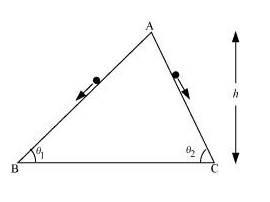Solution.
No; the stone moving down the steep plane will reach the bottom first
Yes; the stones will reach the bottom with the same speed
$v_{\mathrm{B}}=v_{\mathrm{C}}=14 \mathrm{~m} / \mathrm{s}$
$t_{1}=2.86 \mathrm{~s} ; t_{2}=1.65 \mathrm{~s}$
The given situation can be shown as in the following figure: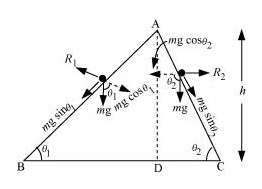Here, the initial height (AD) for both the stones is the same (h). Hence, both will have the same potential energy at point A.
As per the law of conservation of energy, the kinetic energy of the stones at points B and C will also be the same, i. e.,
$\frac{1}{2} m v_{1}^{2}=\frac{1}{2} m v_{2}^{2}$
$v_{1}=v_{2}=v$, say
$m=$ Mass of each stone
$v=$ Speed of each stone at points $\mathrm{B}$ and $\mathrm{C}$
Hence, both stones will reach the bottom with the same speed, $v$.
For stone I:
Net force acting on this stone is given by:
$F_{\text {nel }}=m a_{1}=m g \sin \theta_{1}$
$a_{1}=g \sin \theta_{1}$
For stone II:
$a_{2}=g \sin \theta_{2}$
$\because \theta_{2}>\theta_{1}$
$\therefore \sin \theta_{2}>\sin \theta_{1}$
$\therefore a_{2}>a_{1}$
Using the first equation of motion, the time of slide can be obtained as:
$v=u+a t$
$\therefore t=\frac{v}{a} \quad(\because u=0)$
For stone I:
$t_{1}=\frac{v}{a_{1}}$
For stone II:
$t_{2}=\frac{v}{a_{2}}$
$\because a_{2}>a_{1}$
$\therefore t_{2} Hence, the stone moving down the steep plane will reach the bottom first. The speed (v) of each stone at points B and C is given by the relation obtained from the law of conservation of energy.$m g h=\frac{1}{2} m v^{2}\therefore v=\sqrt{2 g h}\quad=\sqrt{2 \times 9.8 \times 10}\quad=\sqrt{196}=14 \mathrm{~m} / \mathrm{s}$The times are given as:$t_{1}=\frac{v}{a_{1}}=\frac{v}{\mathrm{~g} \sin \theta_{1}}=\frac{14}{9.8 \times \sin 30}=\frac{14}{9.8 \times \frac{1}{2}}=2.86 \mathrm{~s}t_{2}=\frac{v}{a_{2}}=\frac{v}{\mathrm{~g} \sin \theta_{2}}=\frac{14}{9.8 \times \sin 60}=\frac{14}{9.8 \times \frac{\sqrt{3}}{2}}=1.65 \mathrm{~s}$Question 26: A$1 \mathrm{~kg}$block situated on a rough incline is connected to a spring of spring constant$100 \mathrm{~N} \mathrm{~m}^{-1}$as shown in Fig.$6.17$. The block is released from rest with the spring in the unstretched position. The block moves$10 \mathrm{~cm}$down the incline before coming to rest. Find the coefficient of friction between the block and the incline. Assume that the spring has negligible mass and the pulley is frictionless.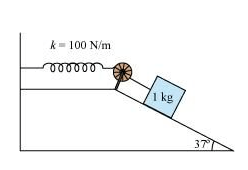Solution. Mass of the block,$m=1 \mathrm{~kg}$Spring constant,$k=100 \mathrm{~N} \mathrm{~m}^{-1}$Displacement in the block,$x=10 \mathrm{~cm}=0.1 \mathrm{~m}$The given situation can be shown as in the following figure.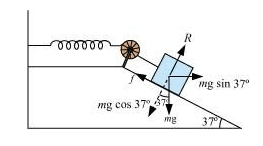At equilibrium: Normal reaction,$R=m \mathrm{~g} \cos 37^{\circ}$Frictional force,$f=\mu R=m \mathrm{~g} \sin 37^{\circ}$Where,$\mu$is the coefficient of friction Net force acting on the block$=m g \sin 37^{\circ}-f=m \operatorname{gsin} 37^{\circ}-\mu m g \cos 37^{\circ}=m g\left(\sin 37^{\circ}-\mu \cos 37^{\circ}\right)$At equilibrium, the work done by the block is equal to the potential energy of the spring, i.e.,$m \mathrm{~g}\left(\sin 37^{\circ}-\mu \cos 37^{\circ}\right) x=\frac{1}{2} k x^{2}1 \times 9.8\left(\sin 37^{\circ}-\mu \cos 37^{\circ}\right)=\frac{1}{2} \times 100 \times 0.10.602-\mu \times 0.799=0.510\therefore \mu=\frac{0.092}{0.799}=0.115$Question 27: A bolt of mass$0.3 \mathrm{~kg}$falls from the ceiling of an elevator moving down with an uniform speed of$7 \mathrm{~m} \mathrm{~s}^{-1}$. It hits the floor of the elevator (length of the elevator$=3 \mathrm{~m}$) and does not rebound. What is the heat produced by the impact? Would your answer be different if the elevator were stationary? Solution. Mass of the bolt,$m=0.3 \mathrm{~kg}$Speed of the elevator$=7 \mathrm{~m} / \mathrm{s}$Height,$h=3 \mathrm{~m}$Since the relative velocity of the bolt with respect to the lift is zero, at the time of impact, potential energy gets converted into heat energy. Heat produced = Loss of potential energy$=m \mathrm{gh}=0.3 \times 9.8 \times 3=8.82 \mathrm{~J}$The heat produced will remain the same even if the lift is stationary. This is because of the fact that the relative velocity of the bolt with respect to the lift will remain zero. Question 28: A trolley of mass$200 \mathrm{~kg}$moves with a uniform speed of$36 \mathrm{~km} / \mathrm{h}$on a frictionless track. A child of mass$20 \mathrm{~kg}$runs on the trolley from one end to the other (10 m away) with a speed of$4 \mathrm{~m} \mathrm{~s}^{-1}$relative to the trolley in a direction opposite to the its motion, and jumps out of the trolley. What is the final speed of the trolley? How much has the trolley moved from the time the child begins to run? Solution. Mass of the trolley, M = 200 kg Speed of the trolley, v = 36 km/h = 10 m/s Mass of the boy, m = 20 kg Initial momentum of the system of the boy and the trolley = (M + m)v = (200 + 20) × 10 = 2200 kg m/s Let v' be the final velocity of the trolley with respect to the ground. Let$v^{\prime}$be the final velocity of the trolley with respect to the ground. Final velocity of the boy with respect to the ground$=v^{\prime}-4$Final momentum$=M v^{\prime}+m\left(v^{\prime}-4\right)=200 v^{\prime}+20 v^{\prime}-80=220 v^{\prime}-80$As per the law of conservation of momentum: Initial momentum = Final momentum$2200=220 v^{\prime}-80\therefore v^{\prime}=\frac{2280}{220}=10.36 \mathrm{~m} / \mathrm{s}$Length of the trolley,$I=10 \mathrm{~m}$Speed of the boy,$v^{\prime \prime}=4 \mathrm{~m} / \mathrm{s}$Time taken by the boy to run,$t=\frac{10}{4}=2.5 \mathrm{~s}\therefore$Distance moved by the trolley$=v^{\prime \prime} \times t=10.36 \times 2.5=25.9 \mathrm{~m}$Question 29: Which of the following potential energy curves in Fig. 6.18 cannot possibly describe the elastic collision of two billiard balls? Here r is the distance between centres of the balls.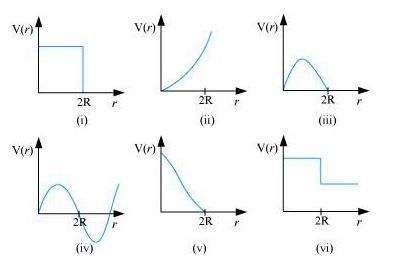Solution. (i), (ii), (iii), (iv), and (vi) The potential energy of a system of two masses is inversely proportional to the separation between them. In the given case, the potential energy of the system of the two balls will decrease as they come closer to each other. It will become zero (i.e.,$\mathrm{V}(r)=0)$when the two balls touch each other, i.e., at$r=2 \mathrm{R}$, where$\mathrm{R}$is the radius of each billiard ball. The potential energy curves given in figures (i), (ii), (iii), (iv), and (vi) do not satisfy these two conditions. Hence, they do not describe the elastic collisions between them. Question 30: Consider the decay of a free neutron at rest:$n \rightarrow p+e^{-}$Show that the two-body decay of this type must necessarily give an electron of fixed energy and, therefore, cannot account for the observed continuous energy distribution in the$\beta$decay of a neutron or a nucleus (Fig. 6.19).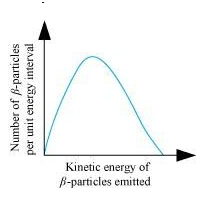[Note: The simple result of this exercise was one among the several arguments advanced by$\mathrm{W}$. Pauli to predict the existence of a third particle in the decay products of$\beta$-decay. This particle is known as neutrino. We now know that it is a particle of intrinsic spin$1 / 2$(like$e^{-}, p$or$n$), but is neutral, and either massless or having an extremely small mass (compared to the mass of electron) and which interacts very weakly with matter. The correct decay process of neutron is:$\left.n \rightarrow p+e^{-+} \mathrm{v}\right]$Solution. The decay process of free neutron at rest is given as:$n \rightarrow p+e^{-}$From Einstein's mass-energy relation, we have the energy of electron as$\Delta m c^{2}$Where,$\Delta m=$Mass defect$=$Mass of neutron$-$(Mass of proton + Mass of electron) c = Speed of light$\Delta m$and$c$are constants. Hence, the given two-body decay is unable to explain the continuous energy distribution in the$\beta\$ -decay of a neutron or a nucleus. The presence of neutrino von the LHS of the decay correctly explains the continuous energy distribution